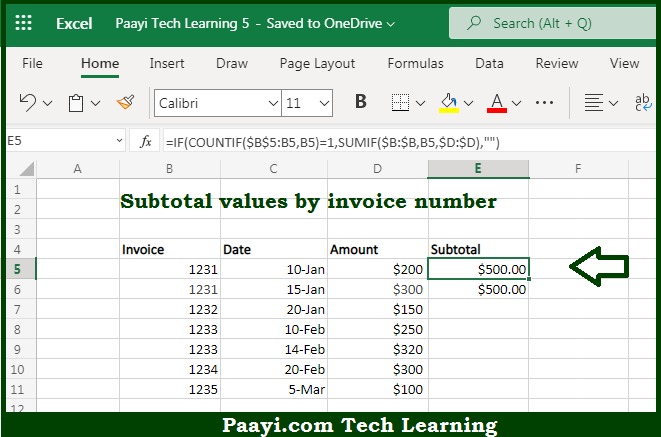# Learn How to SUBTOTAL By Invoice Number in Microsoft Excel

Written by | 0 Comments | 691 Views

In this article, you will learn how to COUNT various things in Microsoft Excel using a single/combination(s) of functions. You will also know how to SUBTOTAL By Invoice Number and see the generic formula.

SUBTOTAL By Invoice Number in Microsoft Excel

The main purpose of this formula is to subtotal values by invoice number. Here we will learn how to subtotal the provided values easily in Microsoft Excel. That implies, with the help of a formula based on the COUNTIF and SUMIF function you can able to subtotal values by invoice number. So, with the help of this formula, you can able to subtotal the provided values easily in Microsoft Excel.

General Formula to SUBTOTAL By Invoice Number

=IF(COUNTIF(range,criteria)=1,SUMIF(range,criteria,sumrange,"")

The Explanation for the SUBTOTAL By Invoice NumberSo we know that with the help of the given formula above you can able to subtotal values by invoice number. Here we will learn how to subtotal the provided values easily in Microsoft Excel. As we know that the above formula uses the COUNTIF function with an expanding range to first check if the current row is the first occurrence of a given invoice number. It should be noted that this expression only returns TRUE when this is the first occurrence of a given invoice number. In our case, the SUMIF function is used to generate a total sum by invoice number, using the amounts in the D column. So, with the help of this formula, you can able to able to subtotal the provided values easily.